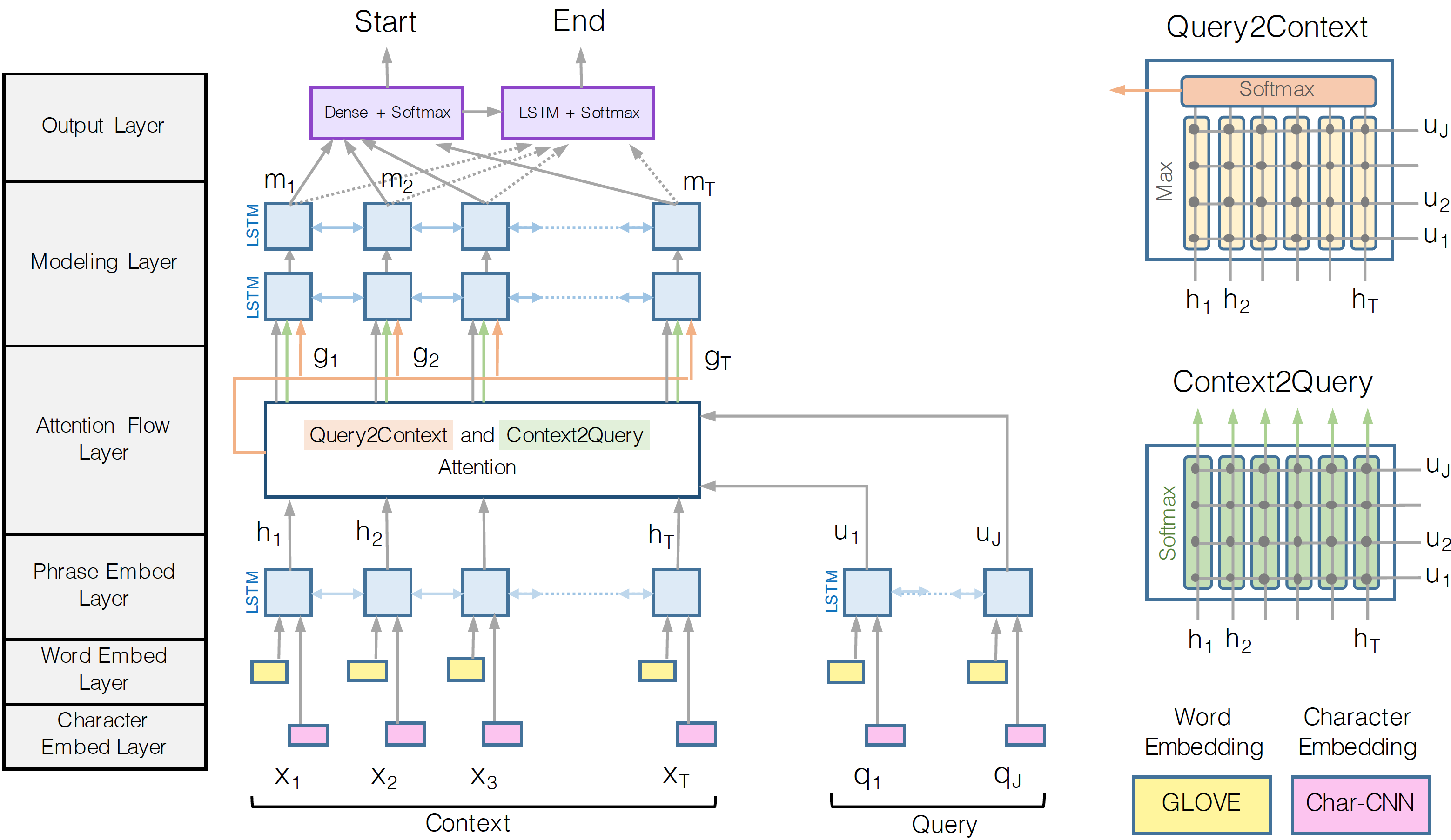## Neural QA

##### September 17, 2018

Another approach to transfer learning in NLP is Question Answering. In the most general case Question Answering is the generation of a textual answer to a given question by a given set of facts in some form. You can find a demo of QA system here

There are many types of this systems:

Categorized by facts representation:

A. Relational database B. Complex data structure - ontology, semantic web, e.t.c. C. Text

1. Yes\No - particular case of matching models
2. Finding bounding indexes for the answer
3. Generate answer by given text and question

Categorized by question type

a. The only possible question - model has no input for questions, it learns to answer only one question defined by training set b. Constant number of questions - model has one-hot encoded input for questions. c. Textual question in special query language - projects like this d. Textual question in free form - model is supposed to some-how encode the text of questions.

For example this article deals with combination C-2-d in this categorization. This combination leads to the necessity of using complex bi-directional attention mechanisms like BiDAF.I, on the contrary, want to concentrate on generating answers without initial markup in the form of answer boundaries. And I will not care about complex question representations, for now. Let’s start with synthetic data baseline as it is described in my previous posts. In this notebook I wrote a list of data generators. Each one is slightly more complicated than the previous one. In the next posts, I will describe my attempts to implement neural network architecture. It should able to generate correct answers for this datasets, starting from the simplest ones.